library(sr)
library(ggplot2)
library(nnet)
library(magrittr)

## Time Series

This vignette will introduce you to selecting good predictive inputs using the Gamma test. There are many situations in which we want to know which variables to measure, in order to predict something else, for example we might want to know which survey questions actually predict voting or buying behavior.

The examples we will use in this vignette all involve determining lags in time series.
How far ahead does a signal appear? If we are trying to predict the future, we are looking for leading indicators, and trying to see how far ahead they lead.
This is a classic example of the problem of figuring out which of our input, predictor variables, is actually predictive. This problem is nonlinear and multivariate. It is the problem that the Gamma test solves.

The vignette will demonstrate using Gamma to - search for causal relationships in time series data, - determine optimum lags and embeddings, and - tune the performance of a neural network.

The vignette will use artificial data sets built from two equations that are commonly used to study chaotic dynamics. Using artificial data will allow us to compare what the Gamma test tells us with what we know is true.

## 1) A chaotic function with no noise

### The Henon Map

Suppose we are asked to analyze this data:


str(henon_x)
#>  num [1:1000] 0.742 1.066 0.486 1.483 -0.654 ...
hix <- data.frame(idx = 1:length(henon_x), x = henon_x)
p <- ggplot(data = hix) +
geom_point(mapping = aes(x = idx, y = x), shape = ".") +
labs(title = "Henon Map") +
xlab("time step") +
ylab("value")
p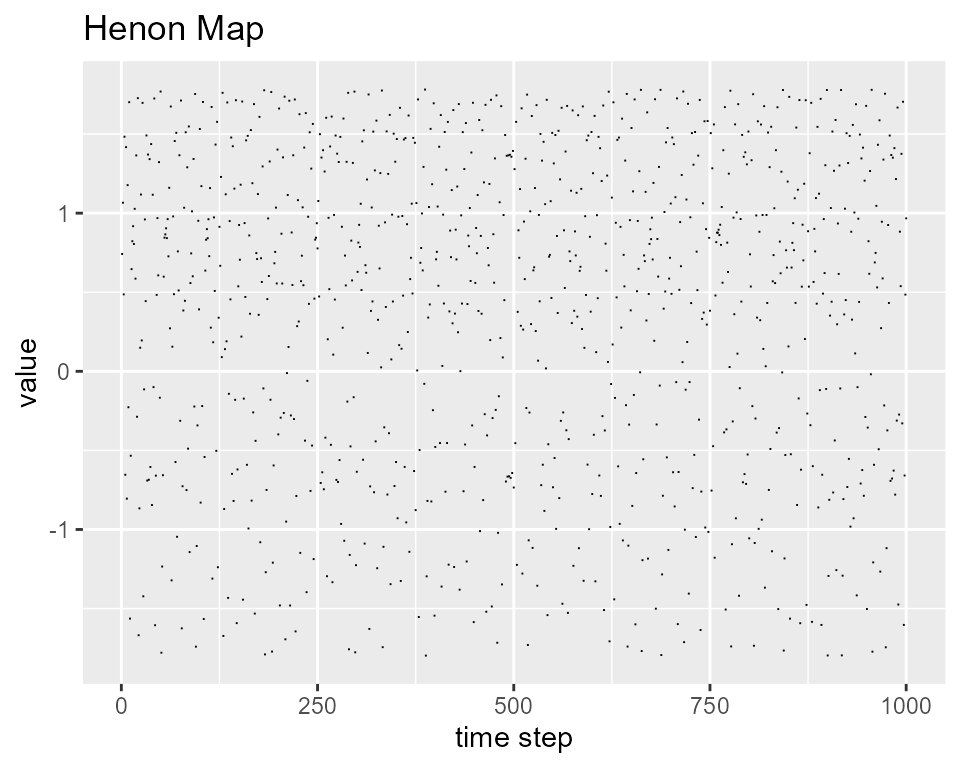It looks a little more regular if we connect the dots.


p + geom_line(mapping = aes(x = idx, y = x), color = "blue")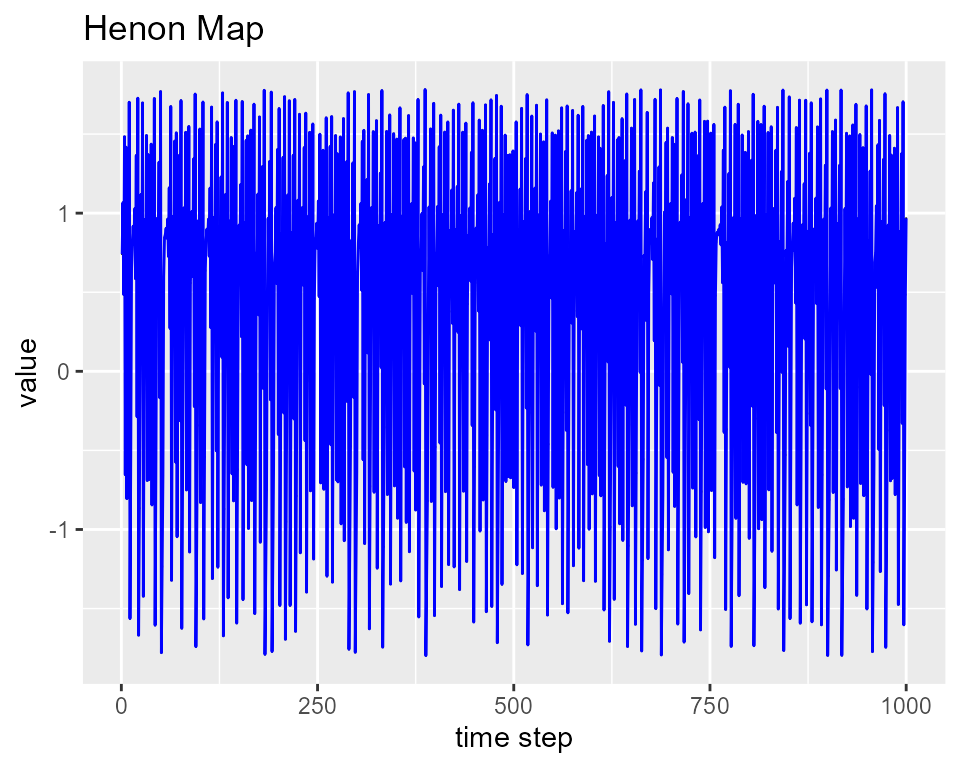Now we can see some structure to it, but it is not at all obvious that it represents a very simple pair of equations. According to Wikipedia: “The Hénon map … is a discrete-time dynamical system. It is one of the most studied examples of dynamical systems that exhibit chaotic behavior. The Hénon map takes a point (x[n], y[n]) in the plane and maps it to a new point

# x[n+1] <- 1 - a * x[n] ^ 2 + y[n]
# y[n+1] <- b * x[n]

For the right choices of the a and b the behavior is chaotic. The data doesn’t look smooth but the underlying functions are - their derivatives are finite. Since y depends only on the previous x, we can predict the next x value from the two previous x values,

### Finding a good embedding

But the overlords who are presenting us with the problem haven’t told us that, maybe they don’t know. It’s not obvious from the graph. How do we figure it out?

We need answers to several questions: - can we predict future values from the past? how well? - how many previous values do we need and which ones? - how good will the resulting model be? When we know the answers to these questions we will know how to train a neural network. We can get the answers from a few simple searches using the Gamma test.

We begin by making a trial embedding, using more inputs than we think we need. I will arbitrarily pick an embedding depth of 15, so we’ll be seeing how well we can predict each point using the previous 15, after the first 15 of course. embed from the stats library reverses the order of the values, so the targets are in column 1, with the lag 1 predictors in column 2.

  search_depth <- 15       # number of candidate predictors
henon_embedded <- embed(as.matrix(henon_x), search_depth+1)  # plus 1 target
targets <- henon_embedded[ ,1]
predictors <- henon_embedded[ ,2:(search_depth+1)]



To find the right embedding, we use an increasing embedding search. This takes the lag 1 predictor and measures Gamma. Then it uses lags 1 and 2, measuring Gamma while adding an additional lag at each step. The result is usually examined visually. The increasing_search function returns a data frame, and plots it unless you set plot = FALSE,

  increasing_search(predictors=predictors,
target=targets,
caption = "henon_x, 15 predictors")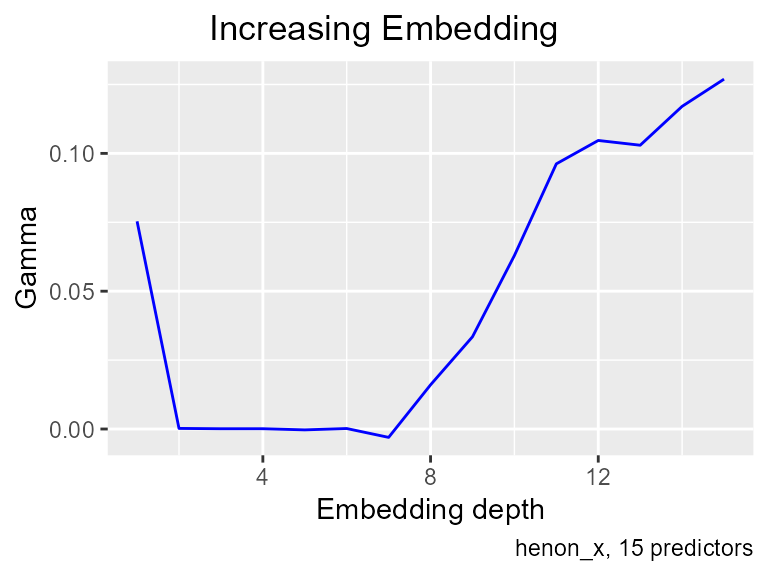Gamma estimates noise - mean squared error. This plot shows Gamma dropping to almost zero with an embedding of 2, in other words, Gamma immediately recognizes the causality in the data. You wont get graphs like this very often with real data. Beyond an embedding of 7, gamma starts to rise again, because the extraneous variables obscure the smooth relationship.

Looking at the graph, one might imagine that an embedding of 6 would be somehow better than 2, “more stable” or whatever. But if the lags between 3 and 6 added any information, Gamma would go down. In this case, of course, they can’t add information, because the mean squared error estimate is already zero. But in general, when you see a long flat floor on the increasing embedding search, the causality is at the beginning. Those additional variables are not confirming votes, it’s just that the relationship is strong enough that it is not obscured by a few random variables.

increasing search also returns its findings as a data.frame:

  tmp <- increasing_search(predictors=predictors,
target=targets,
plot = FALSE)
tmp
#>    Depth         Gamma        vratio
#> 1      1  7.535298e-02  7.385766e-02
#> 2      2  2.146229e-04  2.103639e-04
#> 3      3  9.502454e-05  9.313886e-05
#> 4      4  1.018310e-04  9.981028e-05
#> 5      5 -3.168849e-04 -3.105966e-04
#> 6      6  1.771994e-04  1.736830e-04
#> 7      7 -3.036628e-03 -2.976369e-03
#> 8      8  1.602964e-02  1.571154e-02
#> 9      9  3.346332e-02  3.279927e-02
#> 10    10  6.294790e-02  6.169875e-02
#> 11    11  9.620413e-02  9.429503e-02
#> 12    12  1.046866e-01  1.026091e-01
#> 13    13  1.029603e-01  1.009171e-01
#> 14    14  1.170591e-01  1.147361e-01
#> 15    15  1.269353e-01  1.244164e-01

So I’m going to use an embedding of 2. We will also scale the data before dividing it into training and test sets for the neural network. To build the neural net I will use the nnet package, which expects its data to be scaled between 0 and 1. Gamma does not require scaling.


scale01 <- function(x) {
maxx <- max(x)
minx <- min(x)
return(scale(x,
center = minx,
scale = maxx - minx))
}

henon_scaled <- scale01(henon_x)

search_depth <- 2
henon_embedded <- embed(as.matrix(henon_scaled), search_depth+1)
p <- henon_embedded[ ,2:(search_depth+1)]
t <- henon_embedded[ ,1]
gamma_test(predictors = p, target = t)
#> $Gamma #>  1.579987e-05 #> #>$vratio
#>  0.0001984668

Real noise in the data is zero, the Gamma estimate is 1.58e-05, close enough for statistical work. To understand the results without having to think about how the data is scaled, use the vratio, which is Gamma divided by Var(targets), so it gives the fraction of variance accounted for by estimated noise, and 1 - vratio gives the part accounted for by the unknown smooth function, .9998 in this case.

### Do we have enough data? - the M list

A second question is, how much confidence can we have in Gamma’s estimate of the true noise? Unfortunately, at this time there isn’t any known way to calculate confidence intervals around Gamma, but the M list gives us a basis for a heuristic confidence estimate. With it we can see if Gamma becomes more stable as we add more data. If it doesn’t, we probably don’t have enough data.

The function get_Mlist computes Gamma for larger and larger portions of the data set. What we are looking for in an M list is stability - after a certain point, Gamma should settle to an estimate that doesn’t drift as more data is added.


get_Mlist(predictors = p,
target = t,
caption = "henon_x, 2 predictors")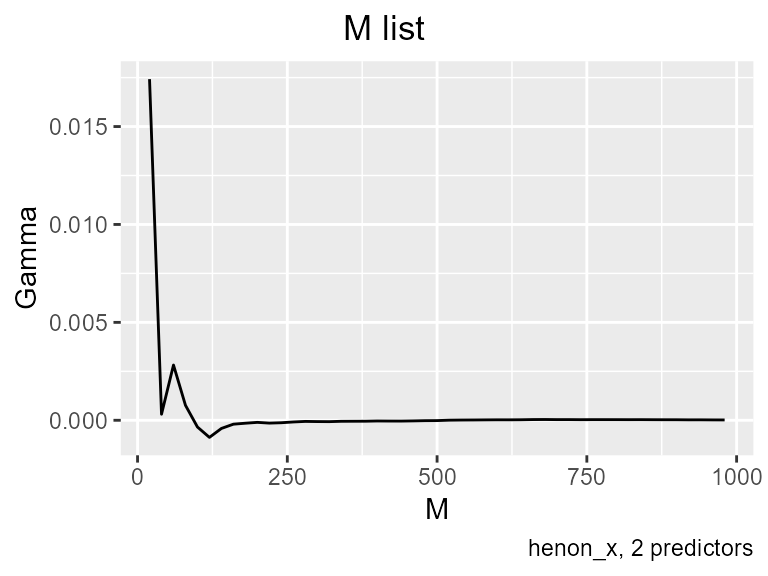In this case - a deterministic function - as M increases Gamma is supernaturally stable. Again, you won’t see graphs like this in the real world. Based on this M list, I’m going to put 600 cases in the training set and keep 400 for the test set.

lt <- length(t)

train_t <- t[1:600]
train_p <- p[1:600, ]

test_t <- t[601:lt]
test_p <- p[601:lt, ]

### Building a model

Now we are going to build a neural net using the nnet package. We will begin by using the parameters on the reference page example, and set the hidden layer size equal to the number of predictors, a bit of bad advice one can find on the internet.


set.seed(3)

n_model <- nnet(x = train_p, y = train_t, size = 2, rang = 0.1,
decay = 5e-4, maxit = 200 )
#> # weights:  9
#> initial  value 56.607699
#> iter  10 value 28.427017
#> iter  20 value 6.741642
#> iter  30 value 1.745130
#> iter  40 value 1.165457
#> iter  50 value 0.902172
#> iter  60 value 0.874990
#> iter  70 value 0.849791
#> iter  80 value 0.847925
#> iter  90 value 0.846205
#> iter 100 value 0.846022
#> iter 110 value 0.845996
#> iter 120 value 0.845991
#> final  value 0.845988
#> converged

predicted_t <- predict(n_model, test_p, type = "raw")

test_result <- data.frame(idx = 1:length(test_t), predicted_t[ ,1], test_t)
colnames(test_result) <- c("idx", "predicted", "actual")

ggplot(data = test_result[1:100, ]) +
geom_line(mapping = aes(x = idx, y = actual), color = "green") +
geom_line(mapping = aes(x = idx, y = predicted), color = "blue") +
geom_line(mapping = aes(x = idx, y = actual - predicted), color = "red") +
labs(y = "predicted over actual",
title = "Henon Model Test, first 100 points")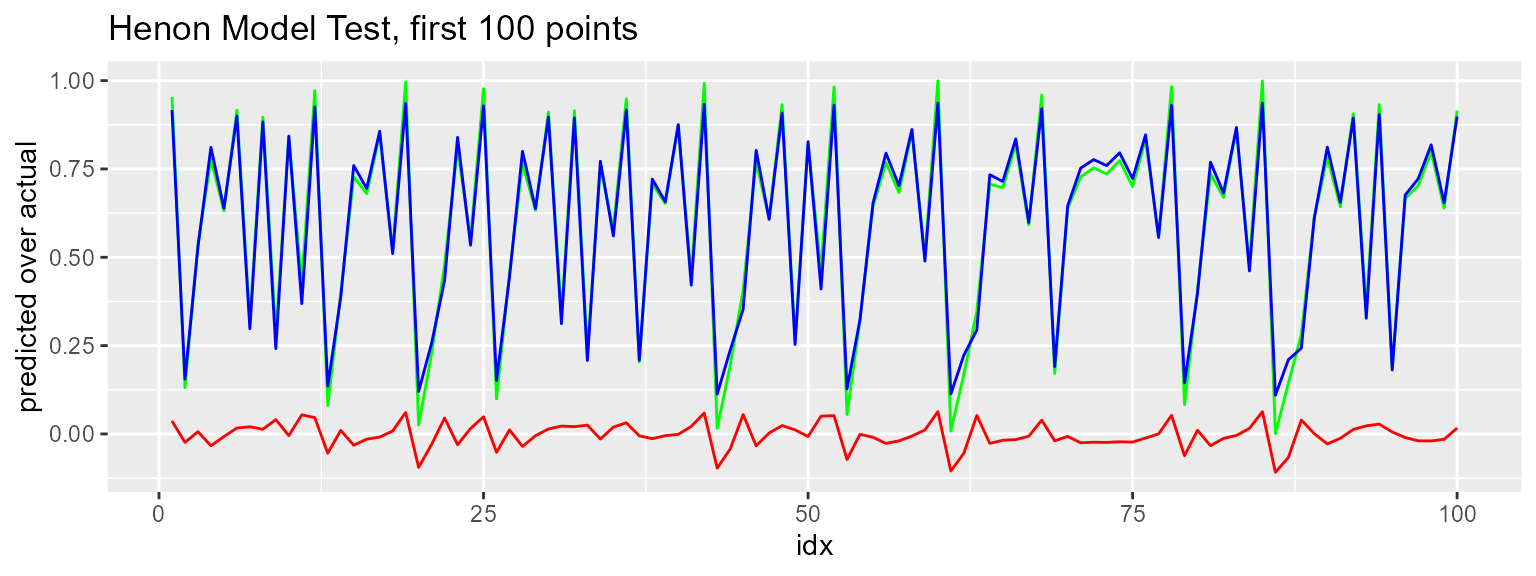For a quick model built with the default parameters, this looks pretty good, but according to Gamma we should be able to to a lot better. The model residuals are .64 and actual error on test data is .50, where Gamma says we should be getting .014.

# Gamma estimate of noise sse
train_gamma <- gamma_test(predictors = train_p, target = train_t)$Gamma train_gamma * length(train_t) #>  0.01405678 # sum of squared error according to model residuals sum(n_model$residuals ^ 2)
#>  0.6469891

# sum of squared errors on test data
with(test_result, sum((actual - predicted)^2))
#>  0.5047026

This vignette is not going to go into detail about working between Gamma and neural net software, that deserves a vignette of its own, but we will make one pass at it here, to show you the general approach. The Gamma test can be used with any neural net software that allows a mean squared error stopping condition for training. nnet allows this with the abstol parameter. We will build a new net using this stopping condition, more hidden layer units, and a smaller decay parameter. Decay is used to prevent over-training, we have the Gamma test which is a more principled way to do that. In general, when you adapt a model to Gamma, you will want to clear away a lot of stuff that’s called “regularization”, much of it is kludges that people have used because they didn’t have a good mean squared error estimator, you do.


estimated_sse <- train_gamma * length(train_t)
gamma_model <- nnet(x = train_p, y = train_t, size = 8, rang = 0.1,
decay = 1e-5, maxit = 2000, abstol =  estimated_sse)
#> # weights:  33
#> initial  value 55.519718
#> iter  10 value 29.883594
#> iter  20 value 3.850031
#> iter  30 value 0.755834
#> iter  40 value 0.636931
#> iter  50 value 0.531718
#> iter  60 value 0.452763
#> iter  70 value 0.393508
#> iter  80 value 0.372772
#> iter  90 value 0.348239
#> iter 100 value 0.327820
#> iter 110 value 0.299960
#> iter 120 value 0.287202
#> iter 130 value 0.273318
#> iter 140 value 0.269654
#> iter 150 value 0.264042
#> iter 160 value 0.247869
#> iter 170 value 0.230083
#> iter 180 value 0.224198
#> iter 190 value 0.212898
#> iter 200 value 0.206761
#> iter 210 value 0.202443
#> iter 220 value 0.199456
#> iter 230 value 0.196864
#> iter 240 value 0.193871
#> iter 250 value 0.192283
#> iter 260 value 0.190241
#> iter 270 value 0.187753
#> iter 280 value 0.187487
#> iter 290 value 0.186336
#> iter 300 value 0.185750
#> iter 310 value 0.185412
#> iter 320 value 0.184559
#> iter 330 value 0.183704
#> iter 340 value 0.182436
#> iter 350 value 0.181801
#> iter 360 value 0.181045
#> iter 370 value 0.180311
#> iter 380 value 0.180067
#> iter 390 value 0.179183
#> iter 400 value 0.176273
#> iter 410 value 0.175255
#> iter 420 value 0.175186
#> iter 430 value 0.174989
#> iter 440 value 0.174703
#> iter 450 value 0.174319
#> iter 460 value 0.173694
#> iter 470 value 0.170974
#> iter 480 value 0.170277
#> iter 490 value 0.167479
#> iter 500 value 0.164335
#> iter 510 value 0.160815
#> iter 520 value 0.154219
#> iter 530 value 0.142478
#> iter 540 value 0.138759
#> iter 550 value 0.138394
#> iter 560 value 0.135955
#> iter 570 value 0.133867
#> iter 580 value 0.131231
#> iter 590 value 0.127103
#> iter 600 value 0.125070
#> iter 610 value 0.122834
#> iter 620 value 0.120273
#> iter 630 value 0.119565
#> iter 640 value 0.119031
#> iter 650 value 0.118655
#> iter 660 value 0.117580
#> iter 670 value 0.117341
#> iter 680 value 0.117332
#> iter 690 value 0.117182
#> iter 700 value 0.117137
#> iter 710 value 0.117032
#> iter 720 value 0.116977
#> iter 730 value 0.116825
#> iter 740 value 0.116582
#> iter 750 value 0.116565
#> iter 760 value 0.116504
#> iter 770 value 0.116465
#> iter 780 value 0.116427
#> iter 790 value 0.116381
#> iter 800 value 0.116326
#> iter 810 value 0.116240
#> iter 820 value 0.116215
#> iter 830 value 0.116177
#> iter 840 value 0.116144
#> iter 850 value 0.116075
#> iter 860 value 0.116017
#> iter 870 value 0.115905
#> iter 880 value 0.115813
#> iter 890 value 0.115798
#> iter 900 value 0.115771
#> iter 910 value 0.115741
#> iter 920 value 0.115550
#> iter 930 value 0.115277
#> iter 940 value 0.114926
#> iter 950 value 0.114861
#> iter 960 value 0.114469
#> iter 970 value 0.114085
#> iter 980 value 0.113647
#> iter 990 value 0.113055
#> iter1000 value 0.111021
#> iter1010 value 0.109730
#> iter1020 value 0.108871
#> iter1030 value 0.107568
#> iter1040 value 0.105111
#> iter1050 value 0.102963
#> iter1060 value 0.099855
#> iter1070 value 0.090529
#> iter1080 value 0.086992
#> iter1090 value 0.081255
#> iter1100 value 0.075932
#> iter1110 value 0.069737
#> iter1120 value 0.067656
#> iter1130 value 0.066020
#> iter1140 value 0.064306
#> iter1150 value 0.064236
#> iter1160 value 0.063839
#> iter1170 value 0.063485
#> iter1180 value 0.063225
#> iter1190 value 0.062781
#> iter1200 value 0.062565
#> iter1210 value 0.062259
#> iter1220 value 0.062015
#> iter1230 value 0.061930
#> iter1240 value 0.061821
#> iter1250 value 0.061686
#> iter1260 value 0.061439
#> iter1270 value 0.061278
#> iter1280 value 0.061212
#> iter1290 value 0.061079
#> iter1300 value 0.060906
#> iter1310 value 0.060819
#> iter1320 value 0.060768
#> iter1330 value 0.060684
#> iter1340 value 0.060498
#> iter1350 value 0.060162
#> iter1360 value 0.060073
#> iter1370 value 0.060008
#> iter1380 value 0.059932
#> iter1390 value 0.059862
#> iter1400 value 0.059743
#> iter1410 value 0.059603
#> iter1420 value 0.059515
#> iter1430 value 0.059439
#> iter1440 value 0.059349
#> iter1450 value 0.059281
#> iter1460 value 0.059186
#> iter1470 value 0.059125
#> iter1480 value 0.058867
#> iter1490 value 0.058817
#> iter1500 value 0.058727
#> iter1510 value 0.058705
#> iter1520 value 0.058598
#> iter1530 value 0.058543
#> iter1540 value 0.058420
#> iter1550 value 0.058310
#> iter1560 value 0.058213
#> iter1570 value 0.058154
#> iter1580 value 0.058117
#> iter1590 value 0.058080
#> iter1600 value 0.058061
#> iter1610 value 0.057962
#> iter1620 value 0.057924
#> iter1630 value 0.057793
#> iter1640 value 0.057621
#> iter1650 value 0.057225
#> iter1660 value 0.056467
#> iter1670 value 0.055204
#> iter1680 value 0.055060
#> iter1690 value 0.054954
#> iter1700 value 0.054831
#> iter1710 value 0.054736
#> iter1720 value 0.054646
#> iter1730 value 0.054560
#> iter1740 value 0.054521
#> iter1750 value 0.054420
#> iter1760 value 0.054396
#> iter1770 value 0.054347
#> iter1780 value 0.054274
#> iter1790 value 0.054245
#> iter1800 value 0.054196
#> iter1810 value 0.054187
#> iter1820 value 0.054142
#> iter1830 value 0.054122
#> iter1840 value 0.054079
#> iter1850 value 0.054066
#> iter1860 value 0.054049
#> iter1870 value 0.054017
#> iter1880 value 0.053994
#> iter1890 value 0.053980
#> iter1900 value 0.053964
#> iter1910 value 0.053948
#> iter1920 value 0.053926
#> iter1930 value 0.053866
#> iter1940 value 0.053817
#> iter1950 value 0.053776
#> iter1960 value 0.053718
#> iter1970 value 0.053657
#> iter1980 value 0.053624
#> iter1990 value 0.053544
#> iter2000 value 0.053508
#> final  value 0.053508
#> stopped after 2000 iterations

predicted_t <- predict(gamma_model, test_p, type = "raw")

test_result <- data.frame(idx = 1:length(test_t), predicted_t[ ,1], test_t)
colnames(test_result) <- c("idx", "predicted", "actual")

ggplot(data = test_result[1:100, ]) +
geom_line(mapping = aes(x = idx, y = actual), color = "green") +
geom_line(mapping = aes(x = idx, y = predicted), color = "blue") +
geom_line(mapping = aes(x = idx, y = actual - predicted), color = "red") +
labs(y = "predicted over actual",
title = "Henon Model Using Gamma")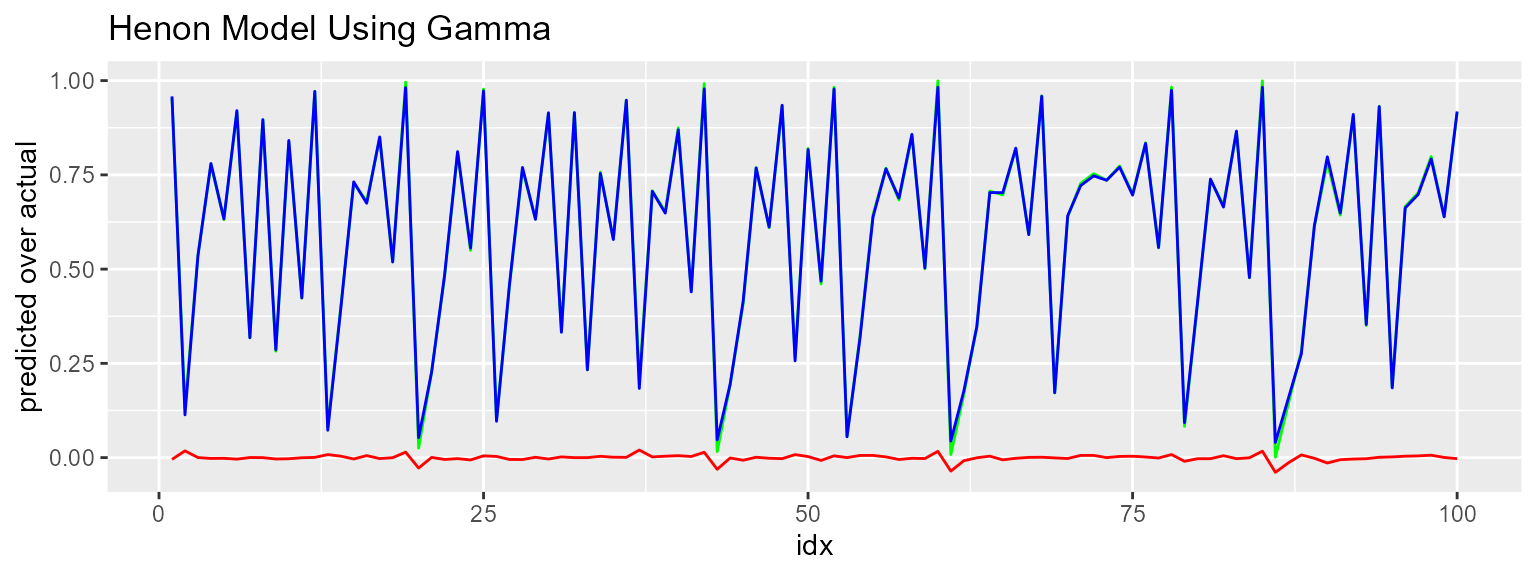# Gamma estimate of error -  gamma times number of observations
estimated_sse
#>  0.01405678

# error according to model residuals
sum(gamma_model$residuals ^ 2) #>  0.03434893 # sum of squared errors on test data with(test_result, sum((actual - predicted)^2)) #>  0.03076592 This does considerably better. Gamma still says we can cut the error in half but I’m not going to fuss around with it. Neural net building is a topic for another vignette, using neural net software that has some documentation and more than one hidden layer. ### Summary: This first example has shown the Gamma test used in four ways: • to identify an embedding - How many previous values of the time series do we want, in order to predict the next one? We saw the Gamma test immediately recognize a causal function even though it was chaotic, and identify the correct embedding using a simple increasing search. • to decide how much data is needed to build a model - The M list tells us how much confidence we can have in Gamma’s estimate. It allows us to make a more informed decision about whether we have enough data, and how much data to use for model training vs. testing. • to evaluate the performance of a model - Although our first model looked OK, given the complexity of what it had to model, the Gamma test told us that we could do better. A mean squared error estimator tells you how well your model should do, so you know when you need to build another model. • to prevent over-training - Learning algorithms are subject to overfitting. Gamma does not work by minimizing some parameter, it estimates the variance of the noise. It is called the Gamma “test” because it directly tests for the presence of a smooth function. This makes it a good complement to machine learning algorithms. ## 2) Relationships with Noise The first example used the Henon Map with no noise, and we saw that Gamma can recognize a deterministic relationship. But what if there’s noise in our data? Going forward we will use a more complex function and put varying amounts of noise on the relationship. As you will see, these examples are not realistic but they are mathematically clear, so you can easily judge the accuracy of the Gamma test. ### Mackey-Glass The function is the Mackey-Glass time delayed differential equation, a chaotic time series that is used to model biological systems. Here’s what it looks like on its own:  str(mgls) #> num [1:4999] 0.274 0.103 0.494 0.725 0.47 ... data.frame(idx = 1:length(mgls), x = mgls) %>% ggplot(data = .) + geom_point(mapping = aes(x = idx, y = x), shape = ".") + labs(title = "mgls raw data")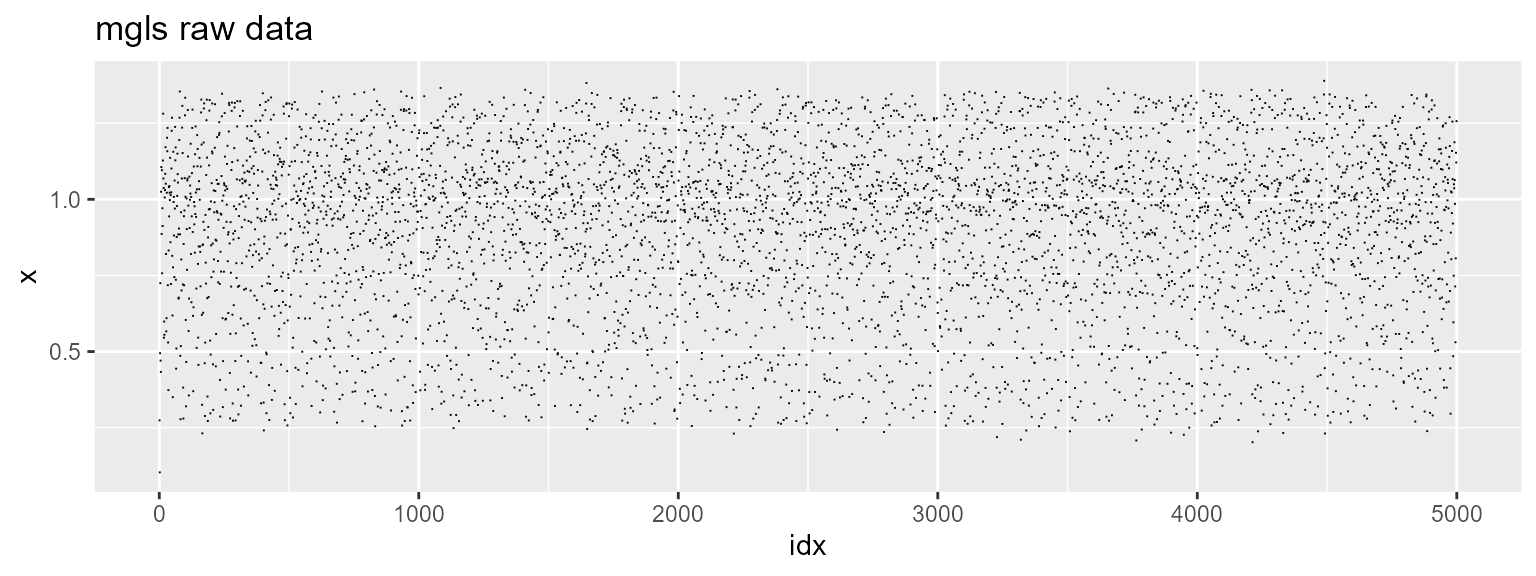This is what chaos looks like. The regular edges show the action of the attractor, but of course they are only clues. We get an increasing search and an M list:  me <- embed(as.matrix(mgls), 13) pre <- me[ ,2:13] tar <- me[ ,1]  increasing_search(predictors=pre, target=tar, caption="mgls, 12 predictors")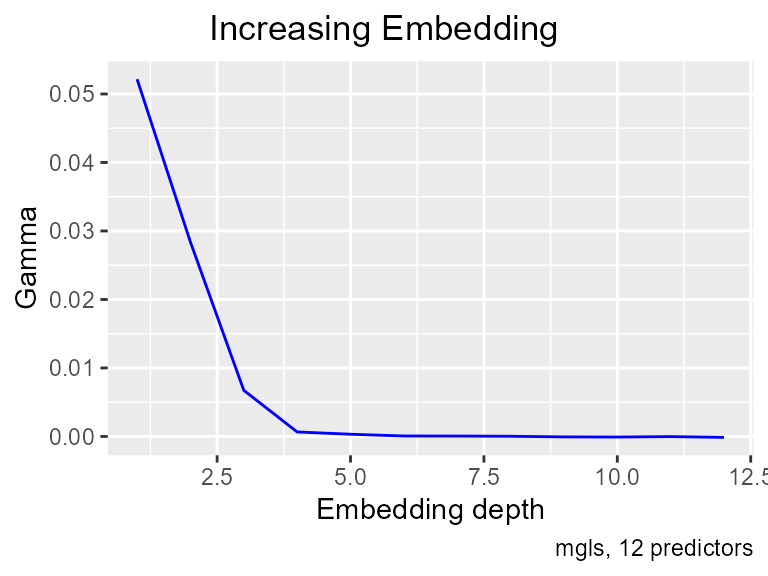get_Mlist(predictors = pre, target = tar, by = 100, caption="mgls, 12 predictors")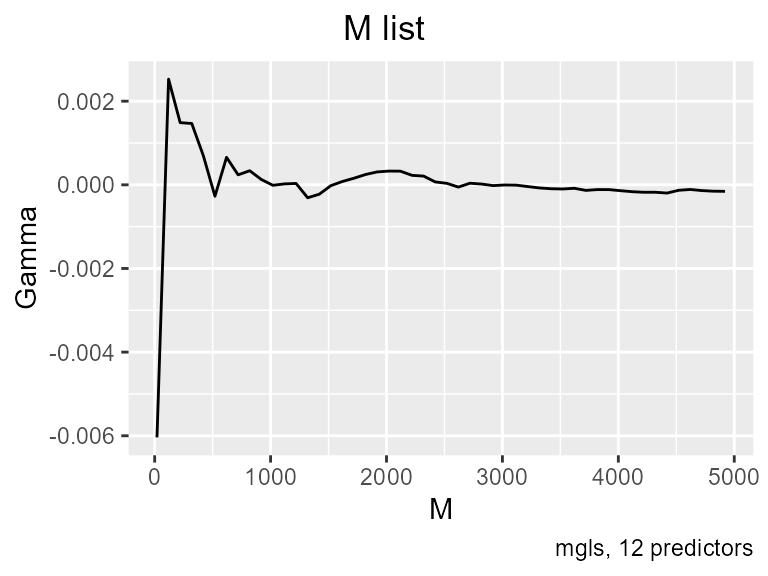This is definitive. The function is in fact driven by the first four lags, and Gamma has shown again that it can reliably detect smooth predictive relationships. ### Mackey-Glass with Noise We’re going to look at two levels of noise, one which is about half the variance of the signal and the second about equal to the signal. We’ll do this in an unnatural way, by adding a random variable to the target only:  # target plus noise with variance .04 t1 <- tar + rnorm(length(tar), mean = mean(tar), sd = sqrt(.04)) # target plus noise with variance .075 t2 <- tar + rnorm(length(tar), mean = mean(tar), sd = sqrt(.075)) # compare these to the variance of the signal vraw <- var(tar) vraw #>  0.07869513 gamma_test(pre, t1) # noise var=.04 added #>$Gamma
#>  0.04130941
#>
#> $vratio #>  0.3435443 gamma_test(pre, t2) # noise var=.75 added #>$Gamma
#>  0.07527744
#>
#> $vratio #>  0.4889851 Gamma’s estimate of noise is quite accurate. We have a lot of data, but the M list on the .075 variance data, when the noise is about half of the signal, shows that Gamma only starts to get stable around a sample size of 4500. #### var = .04 noise  get_Mlist(pre, t1, caption="mgls by 12, .04 noise on output")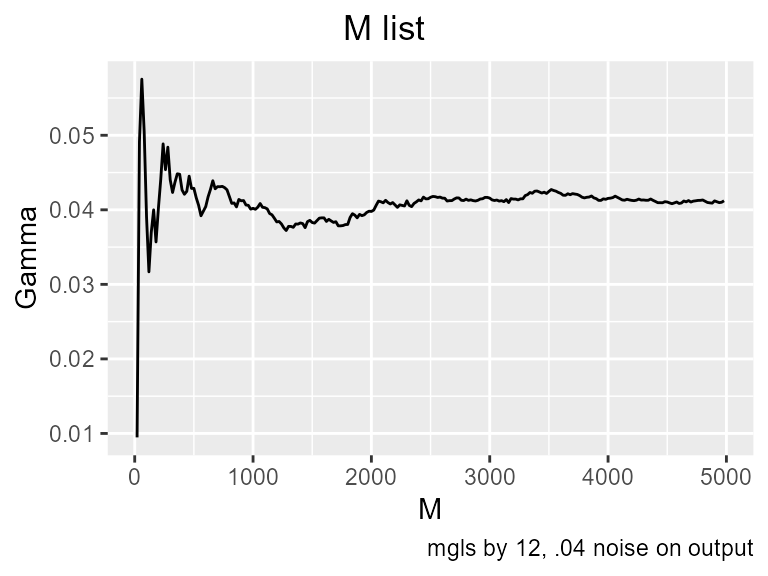#### var = .075 noise  get_Mlist(pre, t2, caption="mgls by 12, .075 noise on output")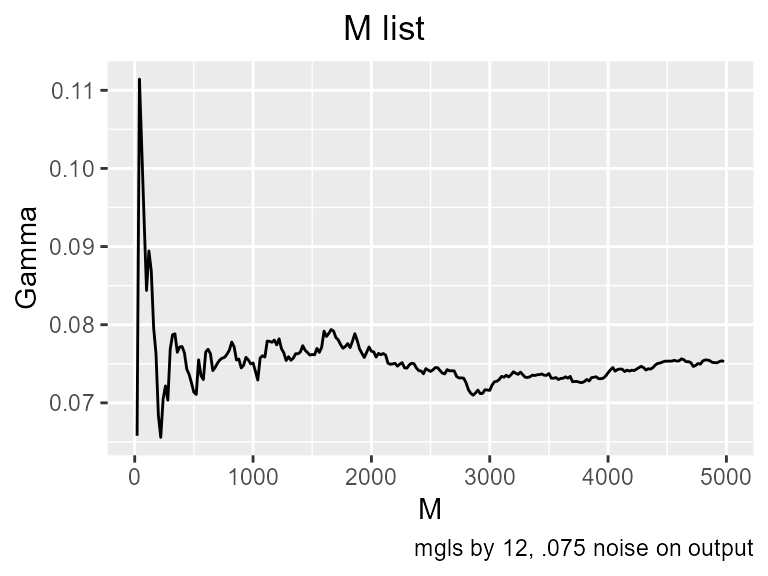These graphs can be displayed in terms of the vratio. This is Gamma divided by the variance of the target, that is, it tells the fraction of variance attributed to noise. .075 is almost equal to the original signal. #### var = .04 noise  get_Mlist(pre, t1, caption="mgls by 12, .04 noise on output", show="vratio")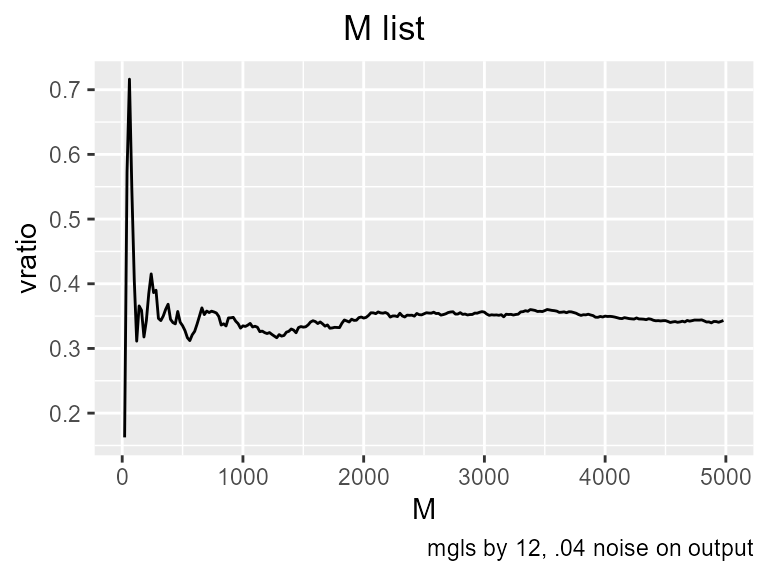#### var = .075 noise  get_Mlist(pre, t2, caption="mgls by 12, .075 noise on output", show="vratio")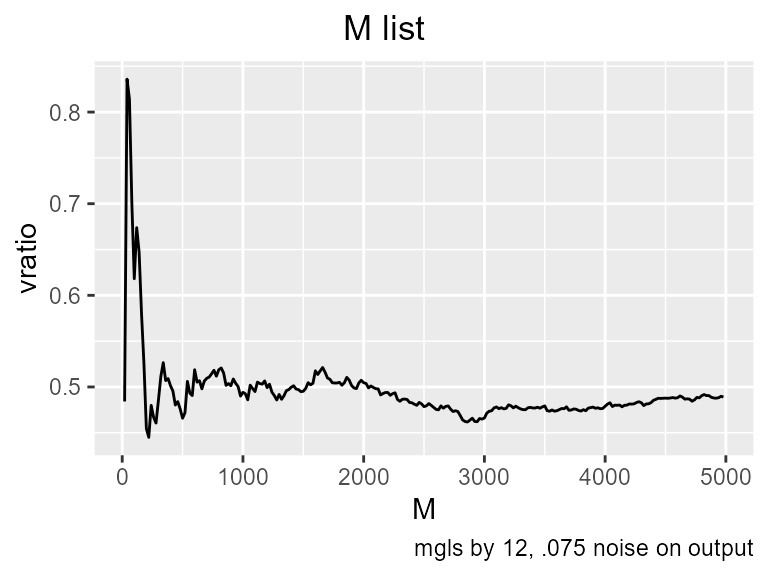You probably have already noticed why I said this is an unrealistic example. I added noise to the target column only. Since the target column is arbitrary based on the embedding, this doesn’t represent a real time series. What it does correspond to is the meaning of the “noise in a relationship”. The t2 model does have an error variance of .075, and that would be the mean squared error of the best neural net we can build on that data. So the usefulness of doing it that way is that we know the answer that the Gamma test is supposed to find. We want to distinguish between the noise on the relationship and the noise in individual measurements. If we add .04 noise to mgls before doing the embedding, we would have noise on both the inputs and the outputs, and the error in the relationship would be somewhere around a sum of the variances of the dimensions in the model. We can see this with a simple example. Suppose we have a function y <- x, and we put.04 noise on both terms. The mean squared error of the prediction will be close to .08.  x <- mgls y <- x + rnorm(length(mgls), mean = mean(mgls), sd = sqrt(.04)) x <- x + rnorm(length(mgls), mean = mean(mgls), sd = sqrt(.04)) mean((y - x) ^ 2) #>  0.08224191 The way these variances combine depends on the function, and if we knew what the function is we wouldn’t be using the Gamma test. So if there is noise on the inputs, even if we put it there, we don’t know how it combines to determine the noise on relationship. Gamma is the only measurement we have of the actual strength of the relationship. mg <- mgls + rnorm(length(mgls), mean = mean(mgls), sd = sqrt(.04)) mge <- embed(mg, 13) pn <- mge[ ,2:13] tn <- mge[ ,1] #### var = .04 noise on all points  increasing_search(pn, tn, caption="mgls by 12, .04 noise on all points")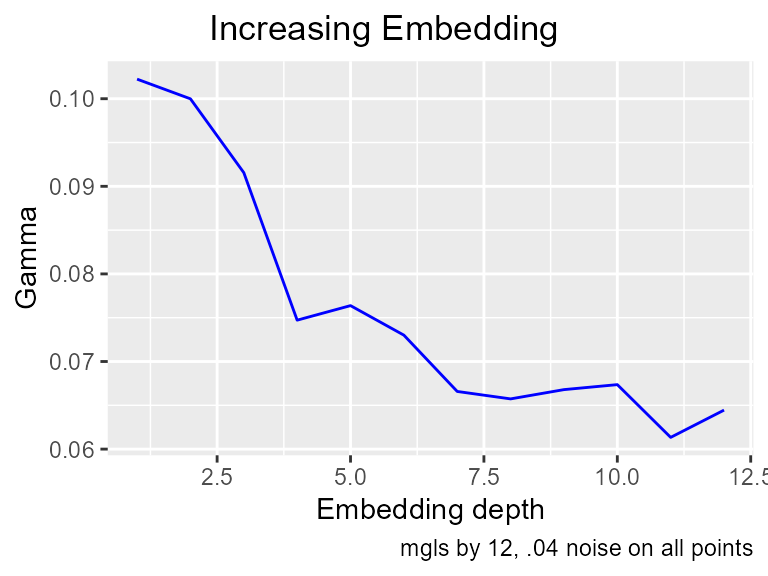#### var = .040 noise on all points  get_Mlist(pn, tn, caption="mgls by 12, .040 noise on all points")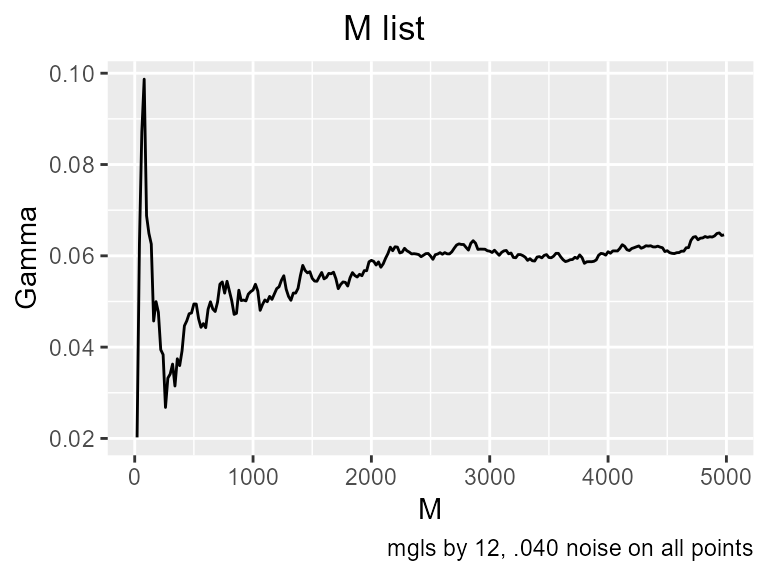gamma_test(pn, tn) #>$Gamma
#>  0.06445131
#>
#>  1.016261
#>
#> $vratio #>  1.020915 The black dots are the distances in output space (y) plotted against distances to near neighbors in the input space (x) . The red dots are the average distances for the first, second, third and so on nearest neighbors. The Gamma regression line in blue is drawn using the squares of those averages. As data density increases, the y intercept of the gamma regression line converges on the noise variance. In this plot we see pure noise. The distances to near neighbors in output space have no relation to the distance in input space, so the Gamma regression line is horizontal. The y intercept is at 1, and the vratio is 1. Gamma is telling us, correctly, that all of the variance in this data comes from noise, the predictors have no smooth relationship with the target. ### Mixed causality and noise: gamma_test(predictors=pre, target=t1, plot = TRUE, caption = "mixed causality and noise")#>$Gamma
#>  0.04130941
#>
#> $vratio #>  0.3435443 This plot uses the .04 noise data set; it shows causality with noise. In the plot, the Gamma regression line has positive slope. This indicates the presence of a smooth relationship because, on average, a point’s first nearest neighbor is closer in output space than its second and third. The intercept is above 0, indicating the presence of noise. The vratio is 0.34, so estimated noise is 34% of the sample variance, and we should be able to build a model with that mean squared error. ### Henon with no noise:  dim <- ncol(henon_embedded) p <- henon_embedded[ ,2:dim] t <- henon_embedded[ ,1] gamma_test(predictors = p, target = t, plot = TRUE, caption = "Deterministic function")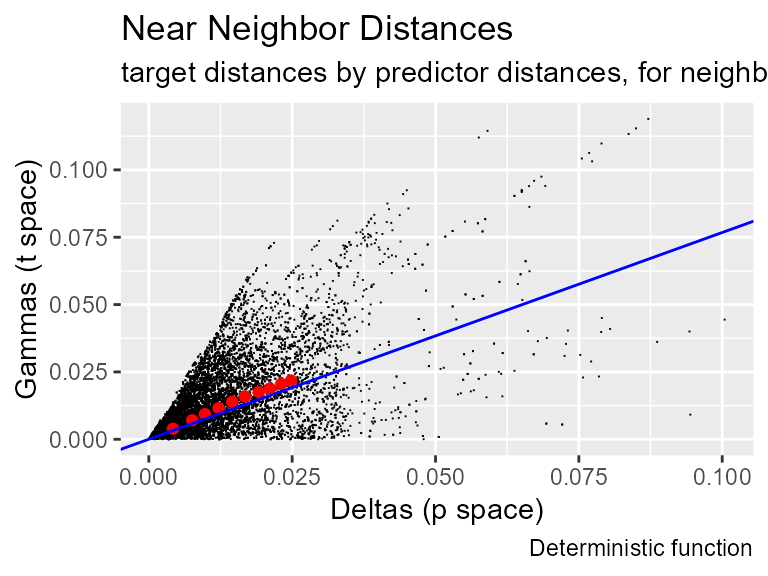#>$Gamma
#>  1.579987e-05
#>
#> \$vratio
#>  0.0001984668

The gamma regression line has positive slope and its intercept is zero, showing that the inputs and output are related by an unknown smooth function. This triangular shape of the delta-gamma graph reflects the curvature of that function. If the function was linear, change in delta would be linearly related to change in gamma, so all of the black points would lie on a line that would intersect the vertex. Instead, because the function has curvature, either the deltas or gammas change more rapidly, producing the spray of points seen here. The trailing lines that fly out to the right represent domains on the surface of the function in which curvature is constant.